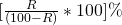Logarithms

It is a passive real number other thanWe write4 then we can writethen we can write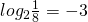Properties of logarithms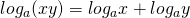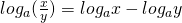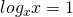Example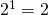so we can write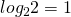Example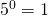in logarithm form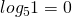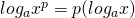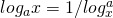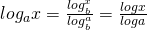Logarithms to the base 10 are known as common logarithms. When base is not mentioned it is taken as 10.
Characteristic – when the number is greater than 1 – the characteristic is one less from the number of digit in the left of the decimal point in the given number.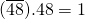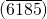̅.41 = 3
When the number is less than 1 one more than the number of zero between the decimal point & the 1st significant digit of the number & it is negative.
0.518 = -1 0.0347 = etc.

Log table-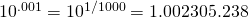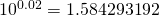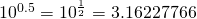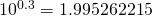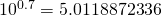Simple and compound interest

Simple interestP= principal amount
R = rate/unit
T = time/year month day etc.

Compound interest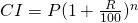P= principal amount
R= rate/unit
T= time unit

Where rate are difference for different unit or time.

Than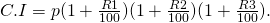Present worth of Rs x lac in year hence is given by present worth=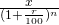Alligation Or Mixture

Alligation- the rate to find the ratio in which two or more ingredients at the given price.must be mixed to produce a mixer of a desired price.
Mean price- the cost price of a unit remobilty of the mix are is called mean price.

Rule of allegation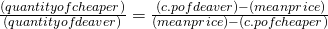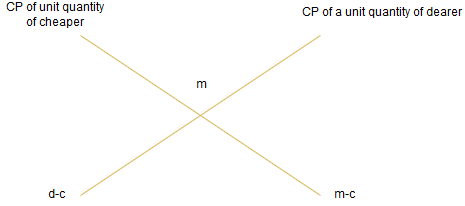cheaper quantity: dearer quantity= (d-m): (m-c)

A container of x unit capacity of liquid, when we taken out some unit say x replaced by water, when we do the same operation n times.
quantity of pure liquid=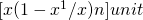Boat and Stream

if the speed of a boat in still water is u km/h & the speed of the streams of v km/h than-
speed towards downstream-Speed towards up stream-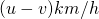if the speed of boat in downstream u km/h and in upstream v km/h
than speed in still water-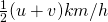rate or speed of stream=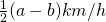Time and Distance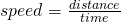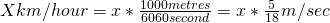If the ratio of the speed of A & B is a:b then the ratio of time taken by them to cover the same distance is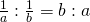“The time taken for covering a certain distance is increasingly proportional to the speed.”
Similarly time taken to finish a work is the inversed proportional to the number of working unit
When by A, cover a certain distance at x km/h & b cover the same distance at y km/h than the average speed of both during the whole journey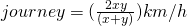Difference distance by two moving body in same direction with ‘u’ m/s and ‘v’ m/s
u>v then their relatives speed = (u-v) m/s
u>v in opposite direction they cross to each other =(With length of a & b meter)
u>v in same direction cross to each other =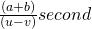if two bodies start at the same time from station A & b to words each other . they have taken time a & b second to reaching A to B & B to A .
then A’s speed: √b:√a

Time and work

If A can do a piece of work in n days then in 1 day’s work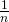If A’s day’s work is 1/n than A can finish the work in n days.

Pipes & cisterns

Inlet- a pipe connected with a tank/cistern that fill it is known as an inlet.
Outlet- a pipe connected with a tank/cistern, emptying it is known as an outlet

1) If a pipe can fill a tank in x hours , then part filled in hour =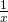2) If pipe can empty a full tank in y hour than part emptied in 1 hour=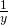3) If a pipe can fill a tank in x hours & another pipe can empty the full tank in y hours. Than an opening both the pipe the net part filled/emptied

Where y<x filled in 1 hour =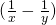Where x>y emptied in 1 hour =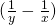part.

Partnership

Partnership
When two or more than two persons are a business jointly they are called partners & the deal is known as partnership.
Ratio of division of gain.
When investments of all the partner are for the same time the gain or loss is distributed among the partners in the ratio of their investment A’s share of profit: B’s share of profit.
When investments are for different time periods then equivalent capital are calculated for unit of time by taking (capital × numbers of units of time) now gain or loss is divided in the ratio of these capitals.
A invest of Rs x for p month & B invest Rs y for q month.
Then profit distributed in xp : yq

Working & sleeping partner
A partner who manages the business is known as working partner & the one who simply invests the money is a sleeping partner.

Ratio and Proportion

Ratio – the ratio of two quantity a & b in the same unit is the fractionand we write it a:b
In a ratio a:b we call a as the first term or antecedent and b the second term or consequent.

Rule– the multiplication or division of each term of a ratio by the same non zero number does not effect the ratio.
Example
4:5 = 8:10= 12:15, also 12:15 = 8:10

Proportion– the quantity of two ratios is called proportion
a:b = c:d we write a:b ::c:d and we say that a,b,c, d, are in proportion. Here a and d are called extremes while b & c are called mean term

Product of means = product of extreme
Thus a:b::c:d
(b×c) = (a×d)

Third proportion
If a:b=b:c, then c is called the third proportion to a & b.
4:8::8:16 – 16 is called third proportion.

Continued proportion e.g. 4:3::8:16
Forth proportion
If a:b = c:d then d is called the fourth proportion to a,b,c

Mean proportion
Mean proportion between a & b is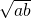4:2::8:16 or 82 = 4×16
8 is mean proportion.

Comparison of ratio

a:b>c:d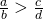Compound ratio– duplicate ratio a:b is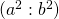Sub duplicate ratio– a:b is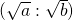Triplicate ratio – a:b isSub triplicate ratio– a:b is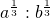If,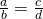thenVerification:-
1) We say that x is directly proportional to y, if x= ky for some constant K
then we write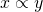2) We say that x is inversely proportional to y, if xy = k for some constant K
then we write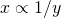Profit and loss

Cost Price– the price of which an article is purchased is called its cost price(CP)
Selling Price– the price at which on article is sold , is called its selling price (SP)
Profit or Gain– if SP is greater than CP , the seller is said to have a profit.

Loss- if SP is less than CP the seller is said to have is called a loss.

Calculation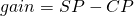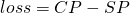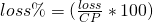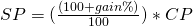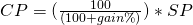If an article is sold at a gain of saythen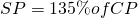If an article is sold at a loss of saythanWhen a person sells two similar items one at a gain of say x% & the other at a loss of x% than the seller always increase a loss given by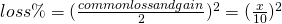If a trader professes to sell his goods at cost price but uses false weight than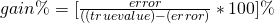Example
If pure ghee costs Rs 100/kg after adulterating it with vegetable oil casting Rs 50/kg a profit of 20% in what ratio does he mix the two.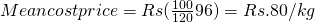Required ratio- 30:20
Oil: ghee

Percentage

To expressas a fraction =Thus,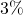==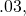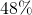=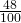==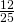To expressas a percentage, we have=Thus=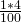== 25%

if the price of commodity increases by R% than the reduction in consumption so as not to increase the expenditure is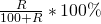Do – decreases R% ______ do__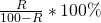Result on Population– let the population of a town be P now & suppose increases at the rate of (@) R % annum then.
Population after n year =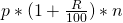Population n year ago =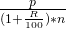Result on depreciation (decrease-) Let the present value of a machine BCp suppose it depreciate @ R% per annum then.
Value of machine after n year =Value of machine n year ago =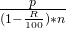if A is R% more than B, B is less than A byIf A is R% less than B, then B is more than A by